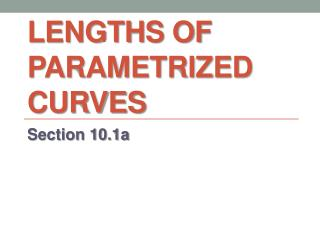DownloadDownload PresentationLengths of parametrized curves

# Lengths of parametrized curves

Download Presentation## Lengths of parametrized curves

- - - - - - - - - - - - - - - - - - - - - - - - - - - E N D - - - - - - - - - - - - - - - - - - - - - - - - - - -
##### Presentation Transcript

1. Lengths of parametrized curves Section 10.1a

2. Definition: Derivative at a Point A parametrized curve , , , has a derivative at if and have derivatives at . The curve is differentiable if it is differentiable at every parameter value. The curve is smooth if and are continuous and not simultaneously zero.

3. Parametric Formula for Recall from Section 3.6: Finding the second derivative:

4. Parametric Formula for Find as a function of if , Step 1: Express in terms of : Step 2: Differentiate with respect to :

5. Parametric Formula for Find as a function of if , Step 3: Divide by :

6. Length of a Smooth Parametrized Curve If a smooth curve , , , is traversed exactly once as increases from to , the curve’s length is (This formula is based on logic similar to that which we used to find the lengths of curves in Section 7.4…)

7. Length of a Smooth Parametrized Curve Find the length of the astroid: First, graph the astroid in [–1.5, 1.5] by [–1, 1] The curve is traced once on this interval. Because of the curve’s symmetry, its length is four times the length of the first quadrant portion…

8. Length of a Smooth Parametrized Curve Find the length of the astroid: Because we’re only working in the first quadrant, we won’t need the absolute value…

9. Length of a Smooth Parametrized Curve Find the length of the astroid: Length of the first quadrant portion of the curve: Length of the entire astroid: Support numerically:

10. Guided Practice Find the length of the given curve.

11. Guided Practice Find the length of the given curve.

12. Guided Practice Find the length of the given curve.

13. Guided Practice Find the length of the given curve.